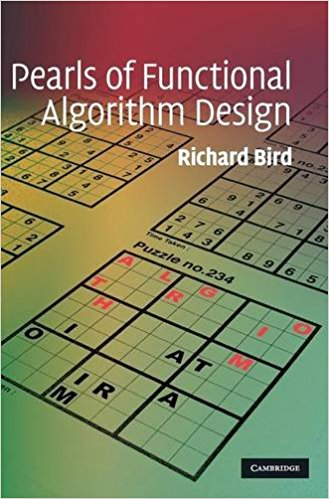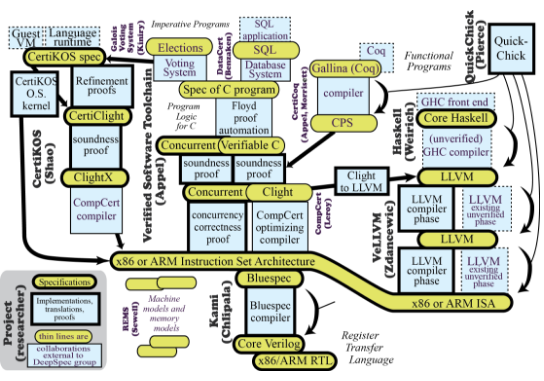## Calculating correct compilers

### Program Calculation and correctness

Project spans two major areas

• Program Calculation
• Formal Verification

## Program Calculation

Calculating the definitions of a function from their specifications## Lets take an example

What does an informal spec looks like?

## An informal spec

``````Imagine a set P of people at a party. Say a subset C of P forms
a celebrity clique if C is nonempty, everybody at the party
knows every member of C, but members of C know only each other.
Assuming there is such a clique at the party and given a binary
predicate "knows" write a program for finding it.``````

## Formalizing the previous spec

``````∅ ⊂ C ⊆ P and

(∀x ∈ P , y ∈ C :: x knows y ∧ (y knows x ⇒ x ∈ C ))``````

``````isClique ps cs  = and [p `knows` c | c <- cs, p <- ps] &&
and [not (c `knows` p) | c <- cs, p <- ps]

cclique ps = head (filter (isClique ps) (subseqs ps))

-- Helper function
subseqs [ ] = [[ ]]
subseqs (x : xs) = map (x:) (subseqs xs) ++ subseqs xs``````

## An example of calculation

`````` -- Base case will be empty
filter (isClique (p : ps)) (subseqs (p : ps))
= {definition of subseqs}
filter (isClique (p : ps)) (map (p:) (subseqs ps) ++ subseqs ps)
= {since filter distributes over ++}
filter (isClique (p : ps)) (map (p:) (subseqs ps)) ++
filter (isClique (p : ps)) (subseqs ps)``````

Further simplification possible but we will be digressing from the main topic

So program calculation provides us with a form of equational reasoning tool

# FORMAL VERIFICATION

## The compcert C compiler## The Deepspec projectCompilers have some of the most in-depth specifications

Examples

• JLS - Java Language Specification
• Ecmascript specification

## Question!

### Can we then calculate a compiler from its specification??

Calculating correct compilers (Bahr and Hutton, 2016)

Hutton's Razor

``````data Expr = Val Int

-- A very simple language``````

A simple evaluator for the language

``````eval :: Expr -> Int
eval (Val n) = n
eval (Add x y) = eval x + eval y``````
``````eval (Add (Val 1) + (Val 2))
= (eval (Val 1)) + (eval (Val 2))
= 1 + 2
= 3``````

Let's calculate a compiler for this language.

## Step 1

We want a stack machine.

In Haskell, a list has stack like semantics: LIFO

So,

``type Stack = [Int]``

Specification:

``````Derive:
evalS :: Expr -> Stack -> Stack

such that
evalS x s = eval x : s                    (1)``````

Val n

`````` evalS (Val n) s
= { specification (1) }
eval (Val n):s
= { definition of eval }
n:s
= {define:pushS n s=n:s}
pushS n s``````

`````` evalS (Add x y) s
= { specification (1) }
= { definition of eval }
(eval x + eval y):s``````

Induction Hypotheses for each element

`````` evalS x s' = eval x : s'

evalS y s' = eval y : s'``````

Proceeding further,

`````` (eval x + eval y):s
= { define: addS (n:m:s) = (m+n):s }
= { induction hypothesis for y }
addS (evalS y (eval x : s))
= { induction hypothesis for x }
addS (evalS y (evalS x s))``````

New stack based evaluator

``````
evalS :: Expr -> Stack -> Stack
evalS (Val n) s = pushS n s
evalS (Add x y) s = addS (evalS y (evalS x s))

pushS :: Int -> Stack -> Stack
pushS n s = n:s

eval :: Expr -> Int
eval x = head (evalS x [])``````

## STEP 2

Stack based machine to Continuation Passing Style

What is Continuation Passing Style (CPS)?

A normal function

``````function add(a, b) {
return a + b;
}``````

The same function CPSed

``````function add_cps(a, b, done) {
done(a + b);
}``````

``````var result = add(1, 2);
// use result here``````

we now have,

``````add_cps(1, 2, function (result) {
// use result here
});``````

Why CPS?

We have

``type Cont = Stack -> Stack``

And specification:

``````Derive
evalC :: Expr -> Cont -> Cont

such that
evalC x c s = c (evalS x s)      (2)``````

Calculating Val n

`````` evalC (Val n) c s
= { specification (2) }
c (evalS (Val n) s)
= { definition of evalS }
c (pushS n s)``````

`````` evalC (Add x y) c s
= { specification (2) }
c (evalS (Add x y) s)
= { definition of evalS }
c (addS (evalS y (evalS x s)))
= { function composition }
(c . addS) (evalS y (evalS x s))
= { induction hypothesis for y from (2) }
evalC y (c . addS) (evalS x s)
= { induction hypothesis for x from (2)}
evalC x (evalC y (c . addS)) s``````

The modified evaluator:

``````evalC :: Expr -> Cont -> Cont
evalC (Val n) c s = c (pushS n s)
evalC (Add x y) c s = evalC x (evalC y (c . addS)) s

evalS :: Expr -> Cont
evalS x = evalC x id``````

## STEP 3

Defunctionalisation (Reynolds, 1972).

Go back two slides and extract the combinators

Substitute the combinators with direct style constructors

``````data Code where
HALT :: Code
PUSH :: Int -> Code -> Code

Substitute and rename the "eval" to "compile" to signify compilation

``````compile :: Expr -> Code
compile x = compile' x HALT

compile' :: Expr -> Code -> Code
compile' (Val n) c = PUSH n c
compile' (Add x y) c s = compile' x (compile' y (ADD c))``````

Compare and contrast!

``````evalS :: Expr -> Cont
evalS x = evalC x id

evalC :: Expr -> Cont -> Cont
evalC (Val n) c s = c (pushS n s)
evalC (Add x y) c s = evalC x (evalC y (c . addS)) s``````

defunctionalised to

``````compile :: Expr -> Code
compile x = compile' x HALT

compile' :: Expr -> Code -> Code
compile' (Val n) c = PUSH n c
compile' (Add x y) c s = compile' x (compile' y (ADD c))``````

Relationship between the two

``````exec :: Code -> Cont
exec HALT       = haltC
exec (PUSH n c) = pushC n (exec c)
``````exec (compile x) s    = evalS x s
exec (compile' x c) s = evalC x (exec c) s``````

Simplifying

``````exec (compile x) s    = eval x : s
exec (compile' x c) s = exec c (eval x:s)``````

We effectively calculated a compiler from its specification!

It uses the Calculus of Constructive Induction.

So it is correct by construction!

### Beyond scope of the presentation

We can fuse the last two steps using a technique called Cutting Out Continuations (Hutton, 2016)

Formalized proofs in Coq: https://github.com/pa-ba/cps-defun

## Research Questions

Can effects be specified?

Can we mechanize the calculation?

Calculating correct compilers (Bahr and Hutton, 2016)

Cutting out continuations (Hutton and Bahr, 2016)